## Euler Circuits

### Learning Outcomes

• Determine whether a graph has an Euler path and/ or circuit
• Use Fleury’s algorithm to find an Euler circuit
• Add edges to a graph to create an Euler circuit if one doesn’t exist
• Identify whether a graph has a Hamiltonian circuit or path
• Find the optimal Hamiltonian circuit for a graph using the brute force algorithm, the nearest neighbor algorithm, and the sorted edges algorithm
• Identify a connected graph that is a spanning tree
• Use Kruskal’s algorithm to form a spanning tree, and a minimum cost spanning tree

In the first section, we created a graph of the Königsberg bridges and asked whether it was possible to walk across every bridge once. Because Euler first studied this question, these types of paths are named after him.

### Euler Path

An Euler path is a path that uses every edge in a graph with no repeats. Being a path, it does not have to return to the starting vertex.

### Example

In the graph shown below, there are several Euler paths. One such path is CABDCB. The path is shown in arrows to the right, with the order of edges numbered.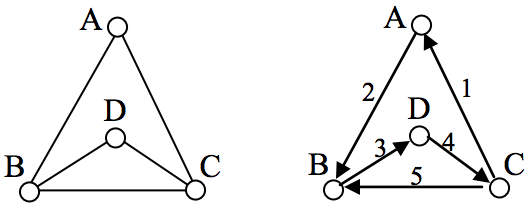### Euler Circuit

An Euler circuit is a circuit that uses every edge in a graph with no repeats. Being a circuit, it must start and end at the same vertex.

### Example

The graph below has several possible Euler circuits. Here’s a couple, starting and ending at vertex A: ADEACEFCBA and AECABCFEDA. The second is shown in arrows.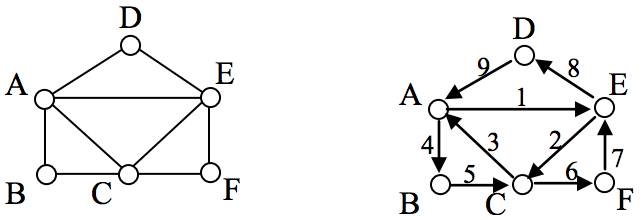Look back at the example used for Euler paths—does that graph have an Euler circuit? A few tries will tell you no; that graph does not have an Euler circuit. When we were working with shortest paths, we were interested in the optimal path. With Euler paths and circuits, we’re primarily interested in whether an Euler path or circuit exists.

Why do we care if an Euler circuit exists? Think back to our housing development lawn inspector from the beginning of the chapter. The lawn inspector is interested in walking as little as possible. The ideal situation would be a circuit that covers every street with no repeats. That’s an Euler circuit! Luckily, Euler solved the question of whether or not an Euler path or circuit will exist.

### Euler’s Path and Circuit Theorems

A graph will contain an Euler path if it contains at most two vertices of odd degree.

A graph will contain an Euler circuit if all vertices have even degree

### Example

In the graph below, vertices A and C have degree 4, since there are 4 edges leading into each vertex. B is degree 2, D is degree 3, and E is degree 1. This graph contains two vertices with odd degree (D and E) and three vertices with even degree (A, B, and C), so Euler’s theorems tell us this graph has an Euler path, but not an Euler circuit.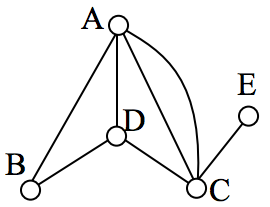### Example

Is there an Euler circuit on the housing development lawn inspector graph we created earlier in the chapter? All the highlighted vertices have odd degree. Since there are more than two vertices with odd degree, there are no Euler paths or Euler circuits on this graph. Unfortunately our lawn inspector will need to do some backtracking.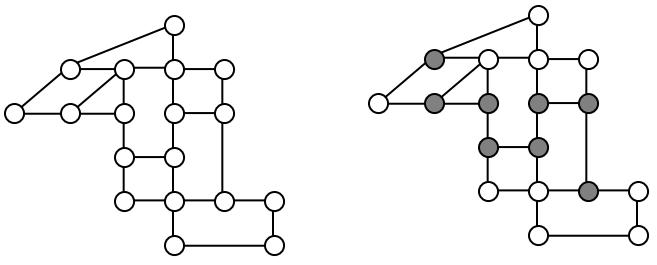### Example

When it snows in the same housing development, the snowplow has to plow both sides of every street. For simplicity, we’ll assume the plow is out early enough that it can ignore traffic laws and drive down either side of the street in either direction. This can be visualized in the graph by drawing two edges for each street, representing the two sides of the street.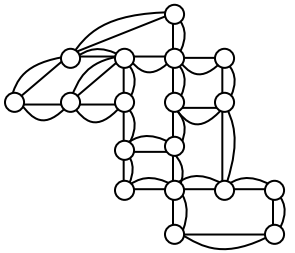Notice that every vertex in this graph has even degree, so this graph does have an Euler circuit.

The following video gives more examples of how to determine an Euler path, and an Euler Circuit for a graph.

### Fleury’s Algorithm

Now we know how to determine if a graph has an Euler circuit, but if it does, how do we find one? While it usually is possible to find an Euler circuit just by pulling out your pencil and trying to find one, the more formal method is Fleury’s algorithm.

### Fleury’s Algorithm

1. Start at any vertex if finding an Euler circuit. If finding an Euler path, start at one of the two vertices with odd degree.
2. Choose any edge leaving your current vertex, provided deleting that edge will not separate the graph into two disconnected sets of edges.
3. Add that edge to your circuit, and delete it from the graph.
4. Continue until you’re done.

The following video presents more examples of using Fleury’s algorithm to find an Euler Circuit.

### Eulerization and the Chinese Postman Problem

Not every graph has an Euler path or circuit, yet our lawn inspector still needs to do her inspections. Her goal is to minimize the amount of walking she has to do. In order to do that, she will have to duplicate some edges in the graph until an Euler circuit exists.

### Eulerization

Eulerization is the process of adding edges to a graph to create an Euler circuit on a graph. To eulerize a graph, edges are duplicated to connect pairs of vertices with odd degree. Connecting two odd degree vertices increases the degree of each, giving them both even degree. When two odd degree vertices are not directly connected, we can duplicate all edges in a path connecting the two.

Note that we can only duplicate edges, not create edges where there wasn’t one before. Duplicating edges would mean walking or driving down a road twice, while creating an edge where there wasn’t one before is akin to installing a new road!

### Example

For the rectangular graph shown, three possible eulerizations are shown. Notice in each of these cases the vertices that started with odd degrees have even degrees after eulerization, allowing for an Euler circuit.

In the example above, you’ll notice that the last eulerization required duplicating seven edges, while the first two only required duplicating five edges. If we were eulerizing the graph to find a walking path, we would want the eulerization with minimal duplications. If the edges had weights representing distances or costs, then we would want to select the eulerization with the minimal total added weight.

### Try It now

Eulerize the graph shown, then find an Euler circuit on the eulerized graph.

### Example

Looking again at the graph for our lawn inspector from Examples 1 and 8, the vertices with odd degree are shown highlighted. With eight vertices, we will always have to duplicate at least four edges. In this case, we need to duplicate five edges since two odd degree vertices are not directly connected. Without weights we can’t be certain this is the eulerization that minimizes walking distance, but it looks pretty good.

The problem of finding the optimal eulerization is called the Chinese Postman Problem, a name given by an American in honor of the Chinese mathematician Mei-Ko Kwan who first studied the problem in 1962 while trying to find optimal delivery routes for postal carriers.  This problem is important in determining efficient routes for garbage trucks, school buses, parking meter checkers, street sweepers, and more.

Unfortunately, algorithms to solve this problem are fairly complex. Some simpler cases are considered in the exercises

The following video shows another view of finding an Eulerization of the lawn inspector problem.